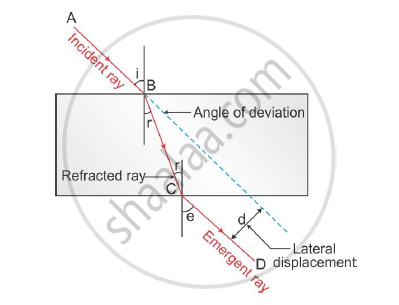# In Your Laboratory You Trace the Path of Light Rays Through a Glass Slab for Different Values of Angle of Incidence (∠i) and in Each Case Measure the Values of the Corresponding Angle of Refraction (∠r) and Angle of Emergence (∠e) - Science

In your laboratory you trace the path of light rays through a glass slab for different values of angle of incidence (∠i) and in each case measure the values of the corresponding angle of refraction (∠r) and angle of emergence (∠e). On the basis of your observations your correct conclusion is:

(a) ∠i is more than ∠r, but nearly equal to ∠e

(b) ∠i is less then ∠r, but nearly equal to ∠e

(c) ∠i is more than ∠e, but nearly equal to ∠r

(d) ∠i is less than ∠e, but nearly equal to ∠r

#### Solution

(a) ∠i is less than ∠r but nearly equal to ∠e.On entering a glass slab, the incident light gets refracted. According to Snell’s law, we get

mu=sini/sinr

For glass μ > 1

∴sinr < sini

or r < i

In refraction of light through a glass slab, the emergent ray is parallel to the incident ray. Thus, ∠i = ∠e.

Concept: Refraction of Light Through a Rectangular Glass Slab
Is there an error in this question or solution?
2015-2016 (March) All India Set 3

Share